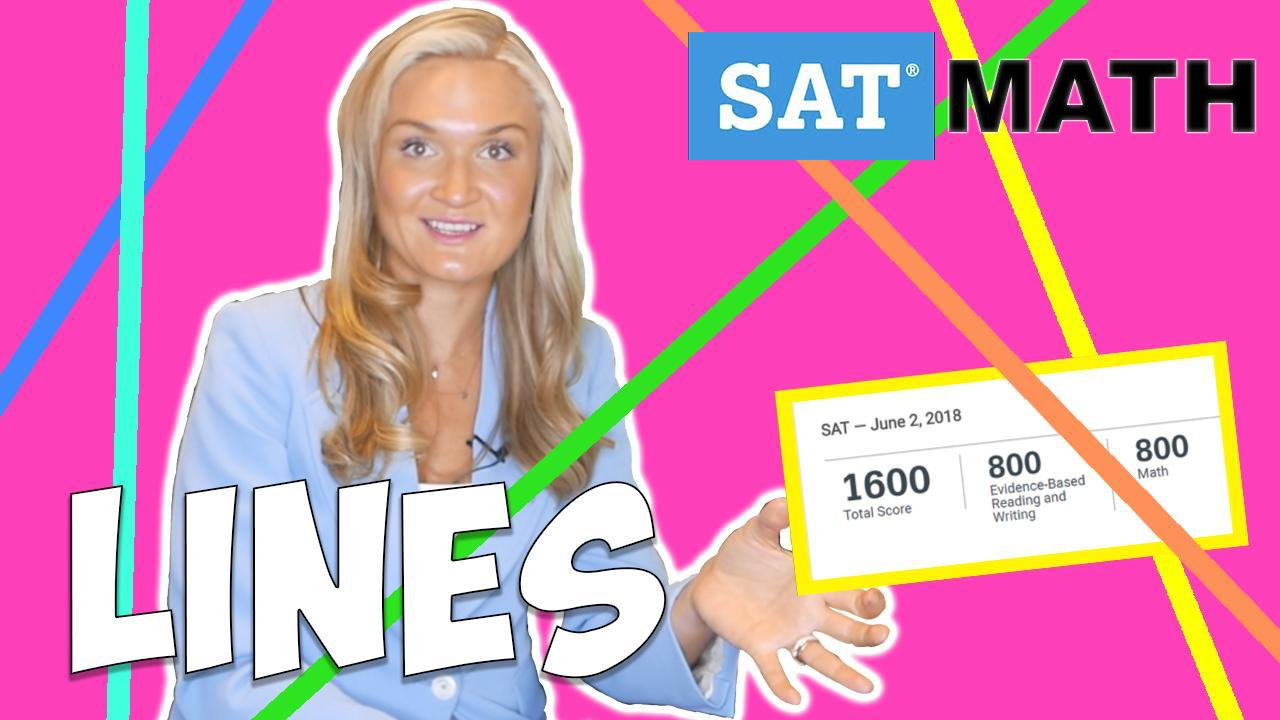How important are lines on the SAT and the ACT?

On the SAT, you should expect to see about 19 questions that are testing your knowledge of lines and linear functions. Considering that there are only 58 math questions in total, lines are responsible for 33% of your math score (about 260 points!). As for the ACT, you should expect to see at least 7 questions based on linear functions. On the ACT test, there are 60 questions in total, and lines are responsible for 12% of your math score or about 4 points.

For my overly confident math prodigies: I realize that you may be very familiar with the lines and linear functions, and you may want to skip this section. Don't rush! I highly encourage you to read through it as the College Board, as well as the ACT, try to disguise simple math problems as something complex and unsolvable. This guide will help you see through the obsurity.

What do linear functions look like😳?

Linear functions can be written in different forms, but the most popular one that you most likely can recognize is a standard form: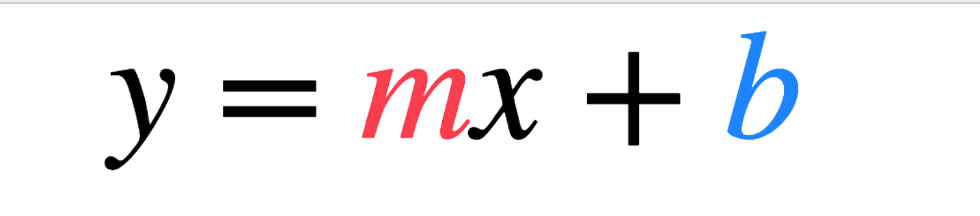where m is a slope, and b is a y-intercept.

If the equation doesn’t exactly look like this - don’t worry.

There a few things that can point to the fact that what you are looking at is a line:

In a linear equation ...

🔴 x and y both have exponents of 1 like in y = 15x + 7

Therefore, if you see ANY exponents in your equation, it’s NOT a line.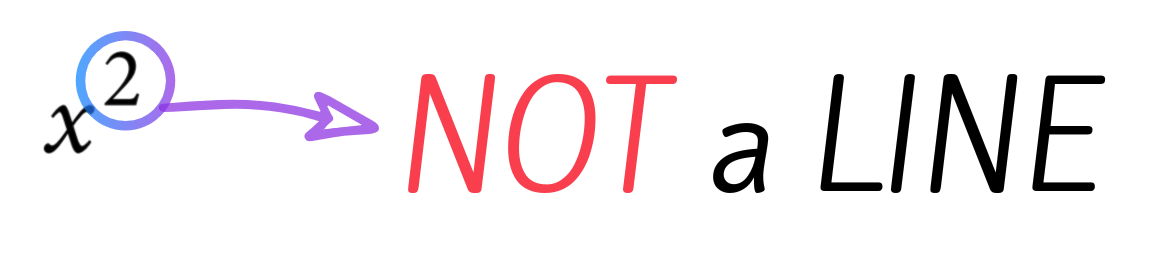🔴 x and y are NOT multiplied together

So if you spot xy hanging out together inseparably, it must NOT be a line.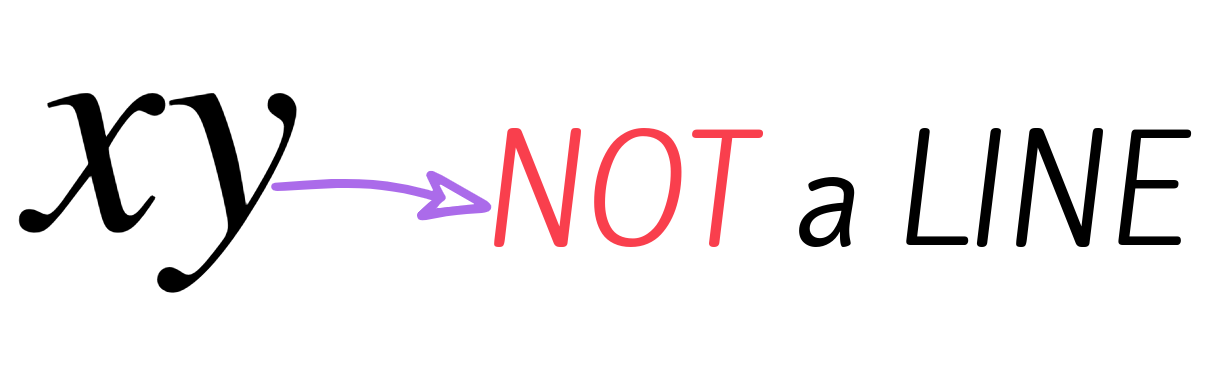🔴 x and y do not appear in denominators, exponents, or radical signs.

So if you see some variables in the denominator, it's very likely that it's NOT a line.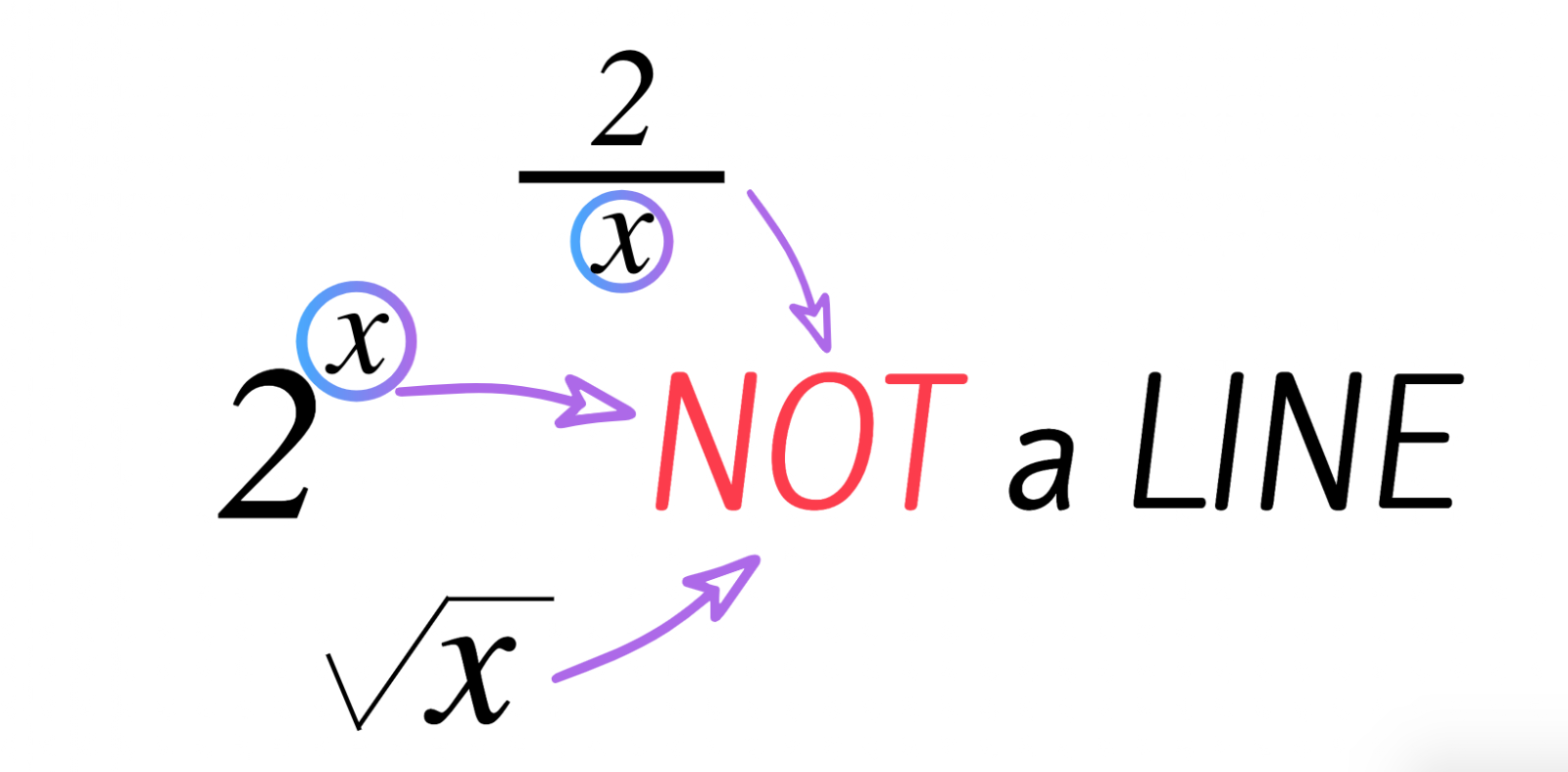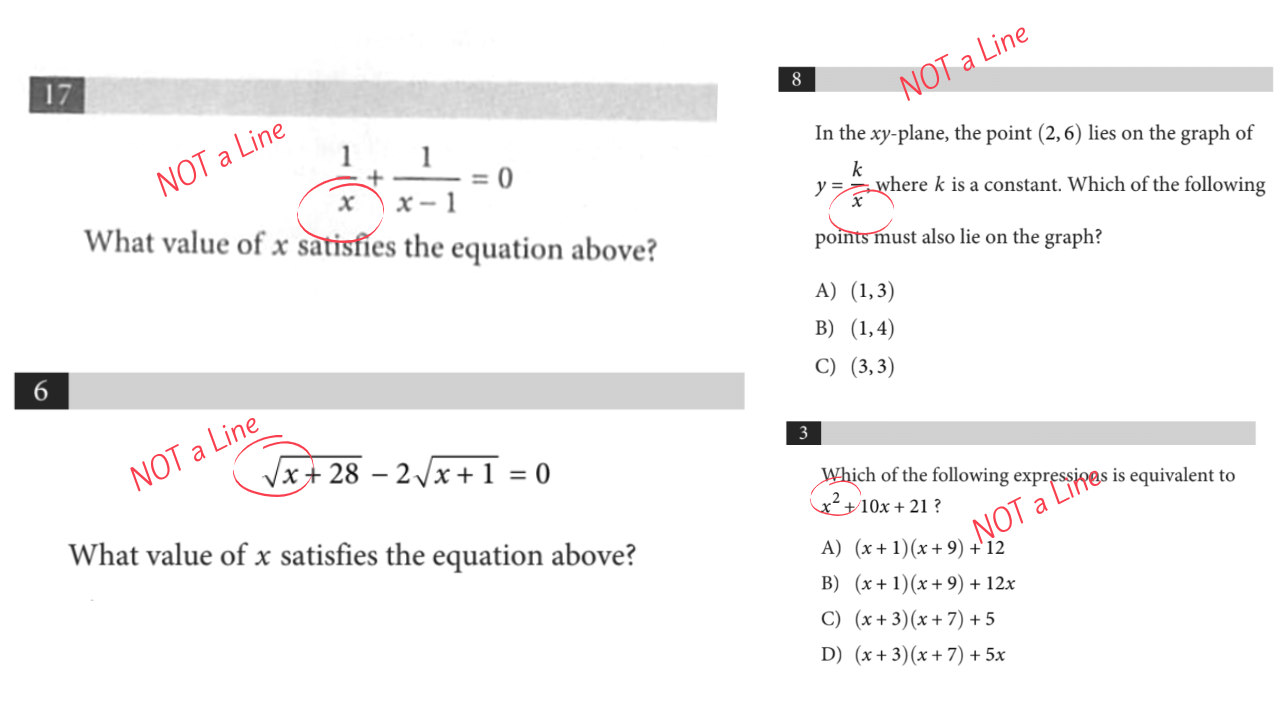Not that you know what is NOT a line, let’s discuss what is a line.

Not that you know what is NOT a line, let’s discuss what is a line.

There are some ways that the test creators can trick you.

For example, when you are looking at the equation below ⬇️⬇️⬇️, your brain usually recognizes that this is a linear equation.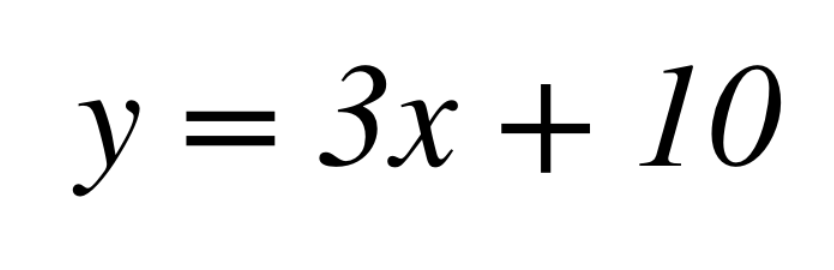However, what do you think about other equations like these:

Believe it or not, but ALL ⬆️these⬆️ equations represent a line. If you simplify them out, you will get a standard linear equation.Remember to always re-write any linear equations into the standard form❗️

Below, you’ll find examples of SAT questions that may not L👀K like a line, but actually are a line.Often, the test will try to trick you by giving you an equation not written in proper form and asking for the slope of the line. Something like this: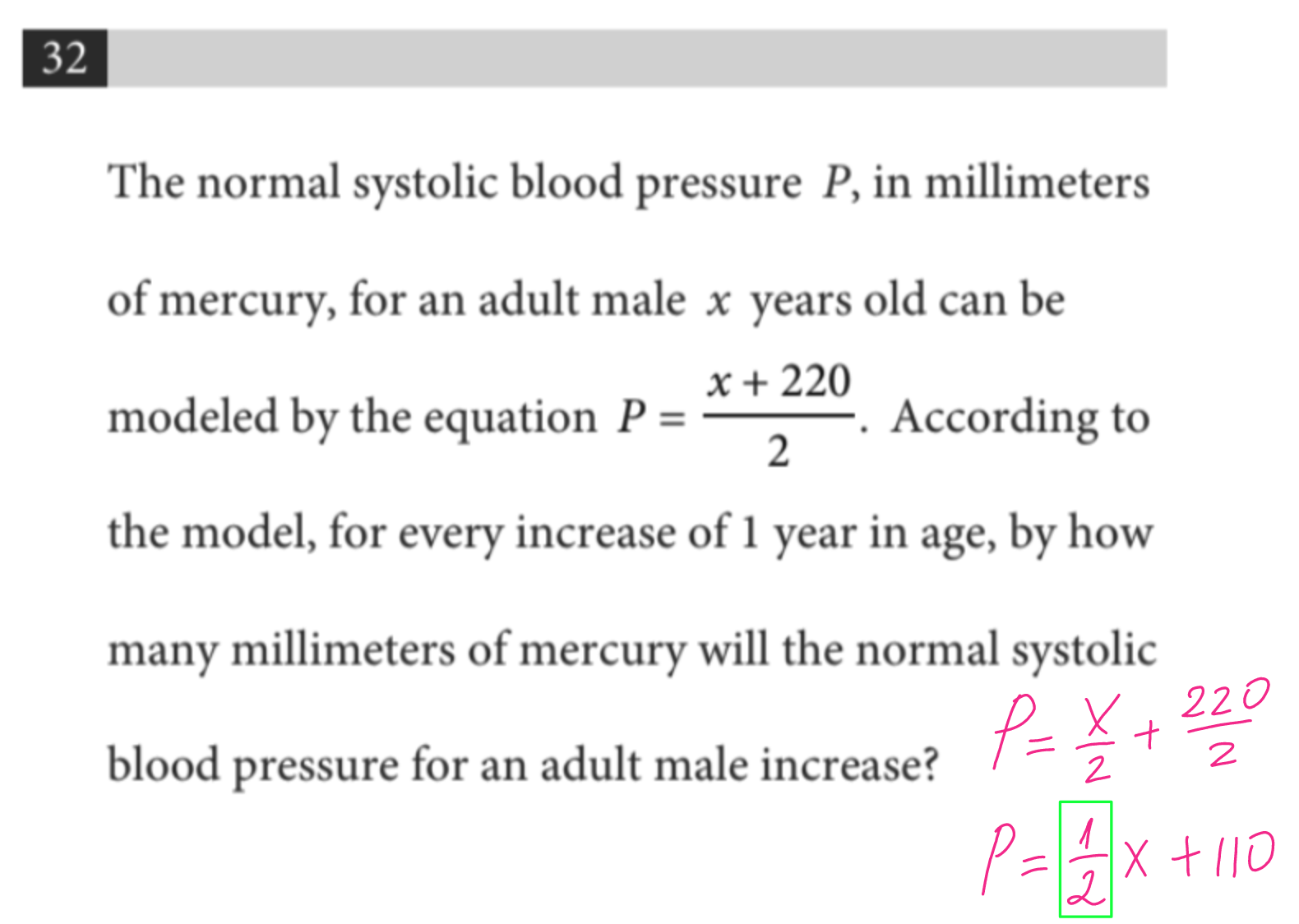Interpreting Linear Functions.

To do well on the SAT and ACT math, you must learn to interpret your functions well. As it turns out, linear functions are often helpful in interpreting real-life scenarios, especially when something is going up or down by a certain number every time. Lines are very helpful because of their slope!

Just a reminder, a slope (m) is a change in Y over the change in X, or “rise over run”. If there is a line given to you, you can calculate a movement over the Y over the movement of X, and this is a slope.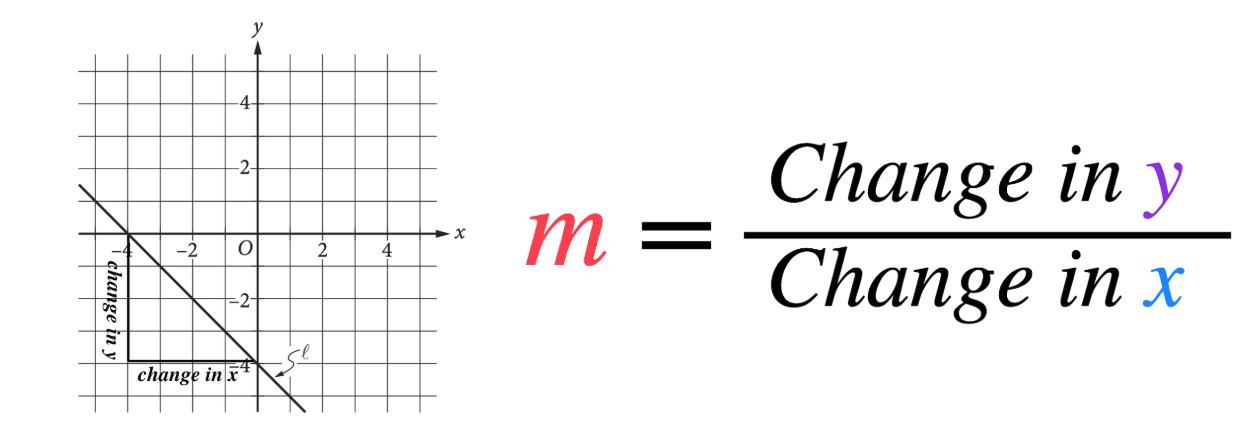What is a slope, really?

A concept of a slope is the amount the y variable changes for an increase in the x value. In other words, it is a rate of change at which y goes up every time x increases by 1. In your example, y = 3x + 10, every time x goes up by 1, y goes up by 3.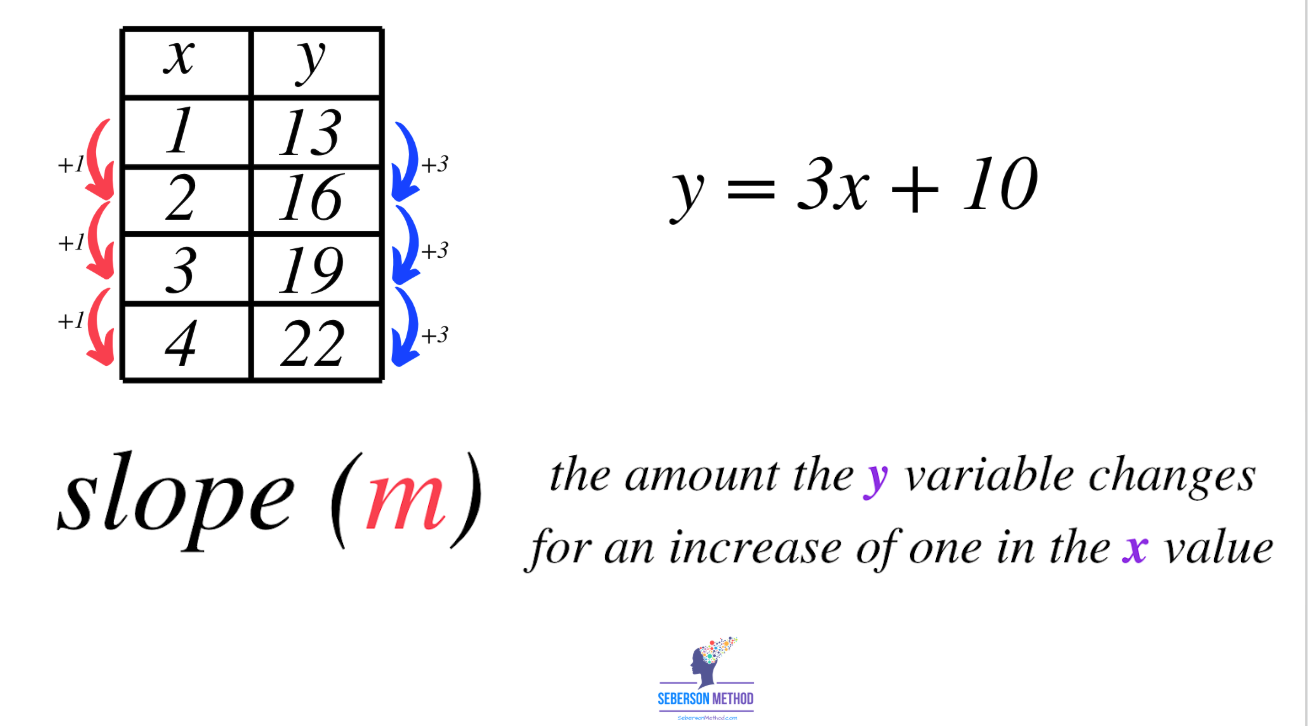Another important component of a linear function is a y-intercept. In many word problems, the Y-intercept (b) represents the initial or starting value or beginning quantity.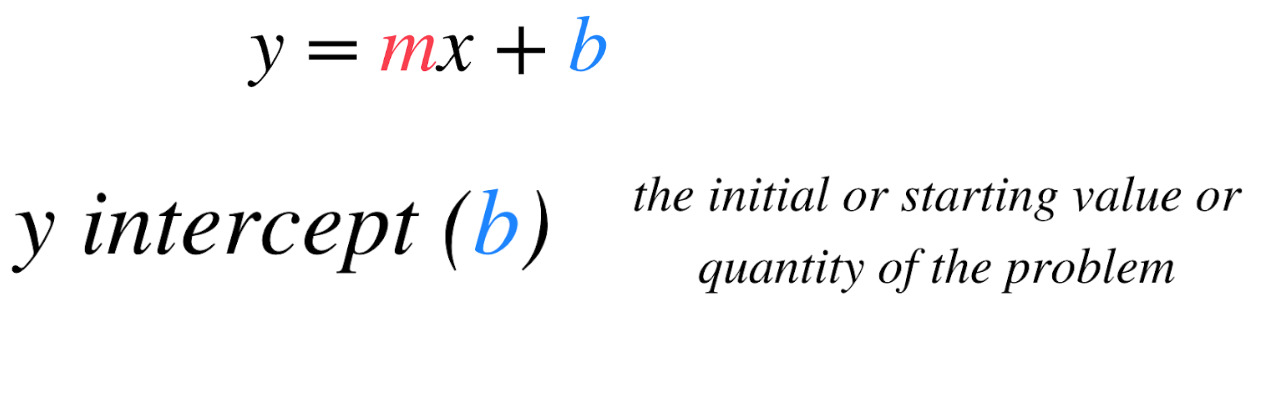Many times, the SAT will ask you to interpret a certain value, like this.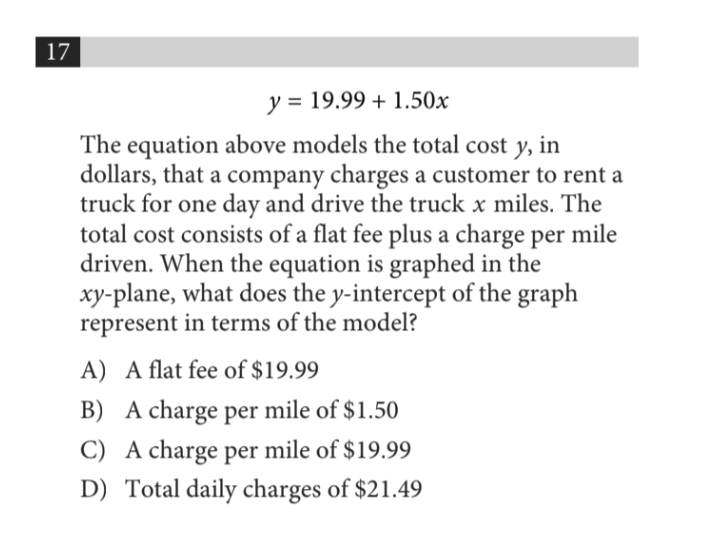The correct answer here comes down to your understanding of the y-intercept. If you said that 19.99 is the initial value, you are 100% correct. Answer choices B, C, and D all represent a slope or a rate.

The SAT may also ask you to interpret the significance of a y-intercept or an x-intercept. For example: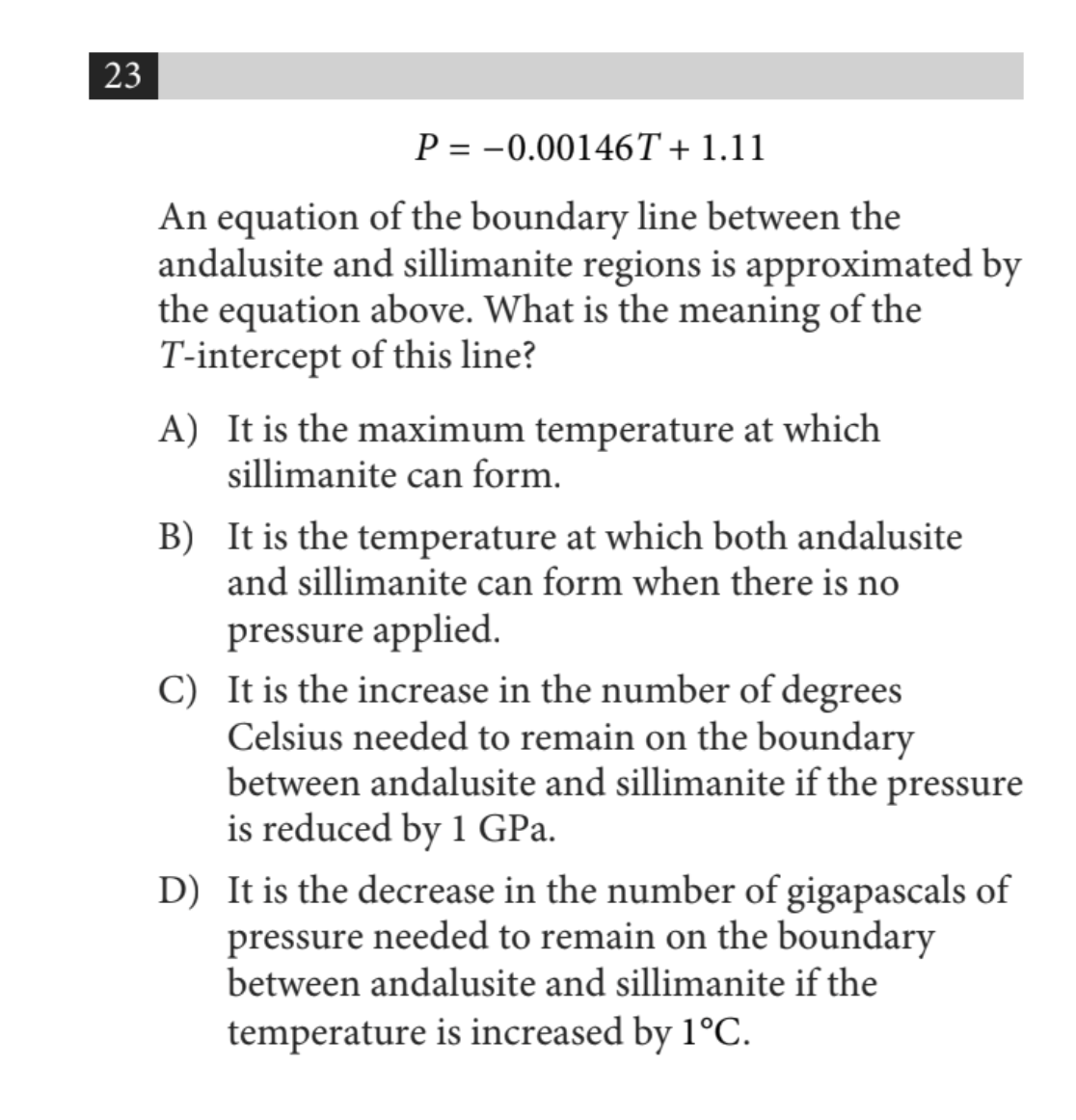Initially, this question may look very confusing, but FEAR NOT! All you need to do is recognize that the equation in question is a line where your T is X and P is Y.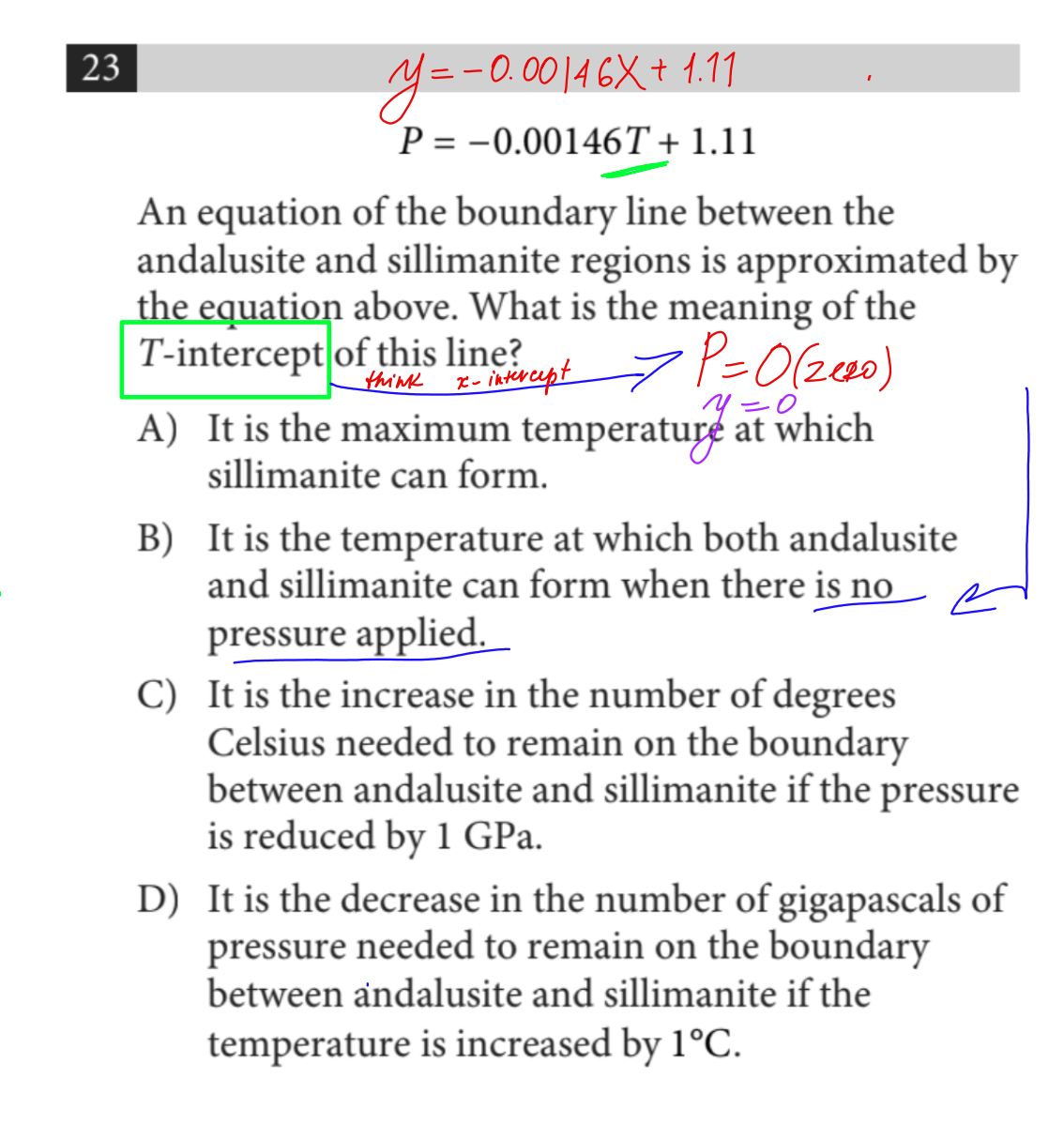FOUR Clear Telltale signs you are looking at a WORD Problem.

Word problems usually involve lines, but it’s especially important to quickly identify whether you are dealing with a linear function or not.

There are some telltale signs that may appear in the word problem that would let you know that what you are looking at is a line or a linear relationship. Here are those words:

⭕ at a constant rate

⭕ rate

⭕ per

⭕ each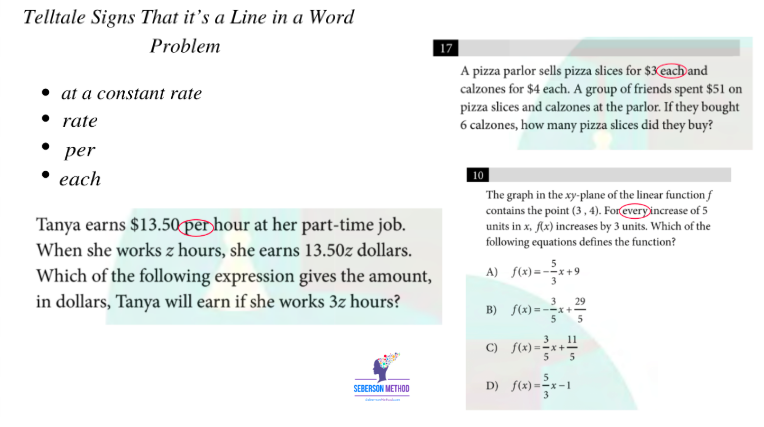When deciding between exponential and linear relationships, quickly scan the problem for these four words.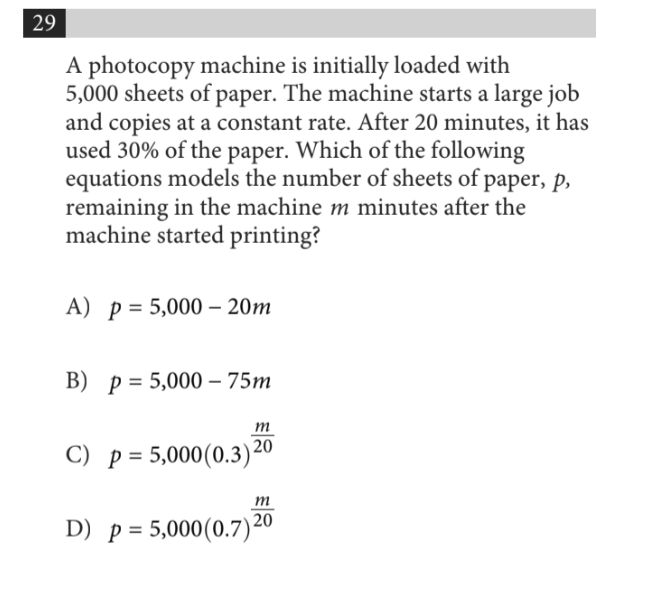In order to decide which function it is, simply look for the keywords. In the third line, you clearly see the phrase “at a constant rate” which clearly outlines a linear relationship. You can then create two points [ (0, 5000) and (20, 3500) ] and find a slope of - 75.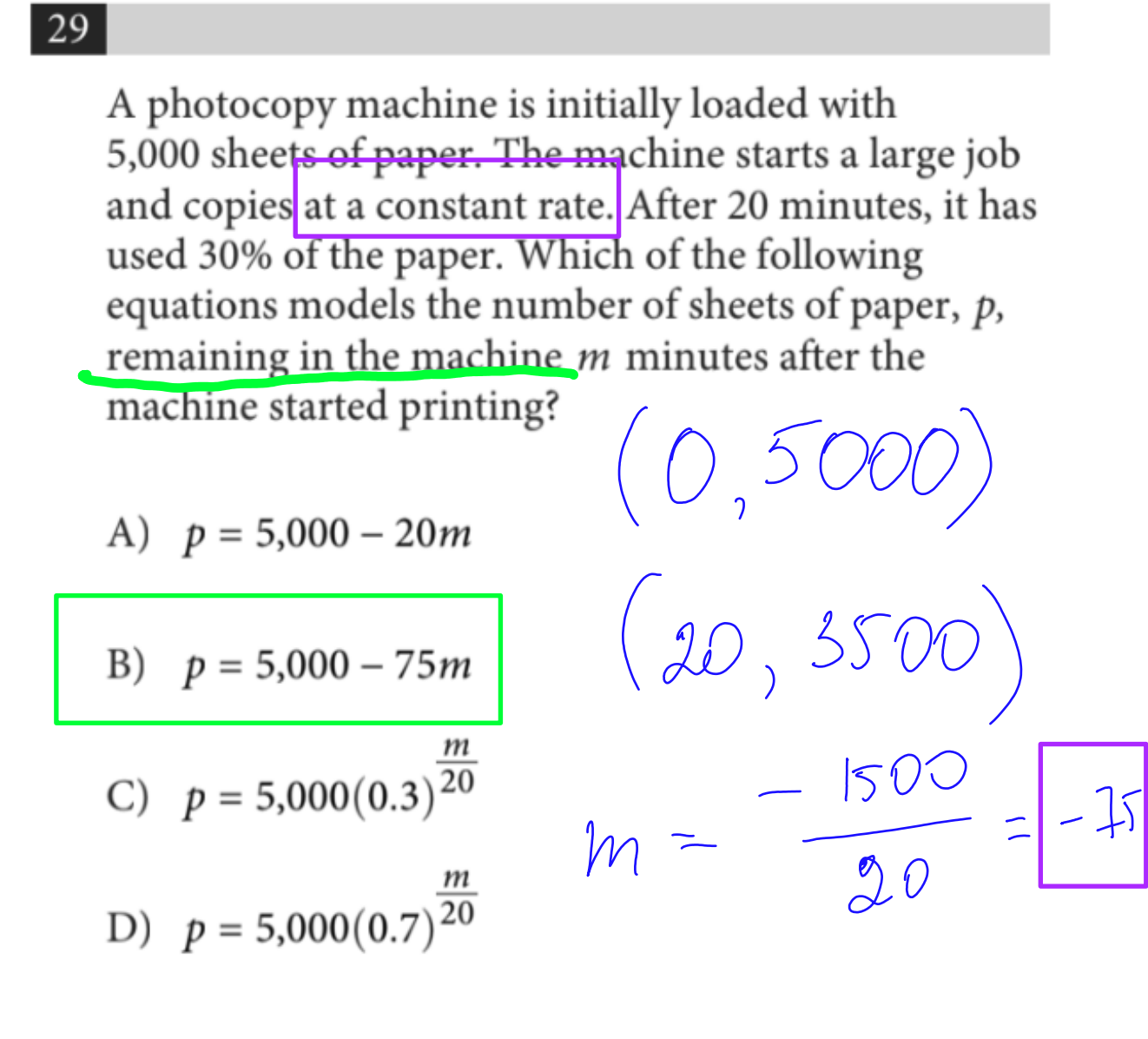Creating a Linear equation from a point and a slope.

If you are given a slope and a point, you can just use a point-slope formula to find an equation of a line.

👉Point-Slope Formula

Another form of a linear equation is known as a point-slope form. Sometimes, you will be given a point-slope form in the answer choices, so make sure you can recognize this form.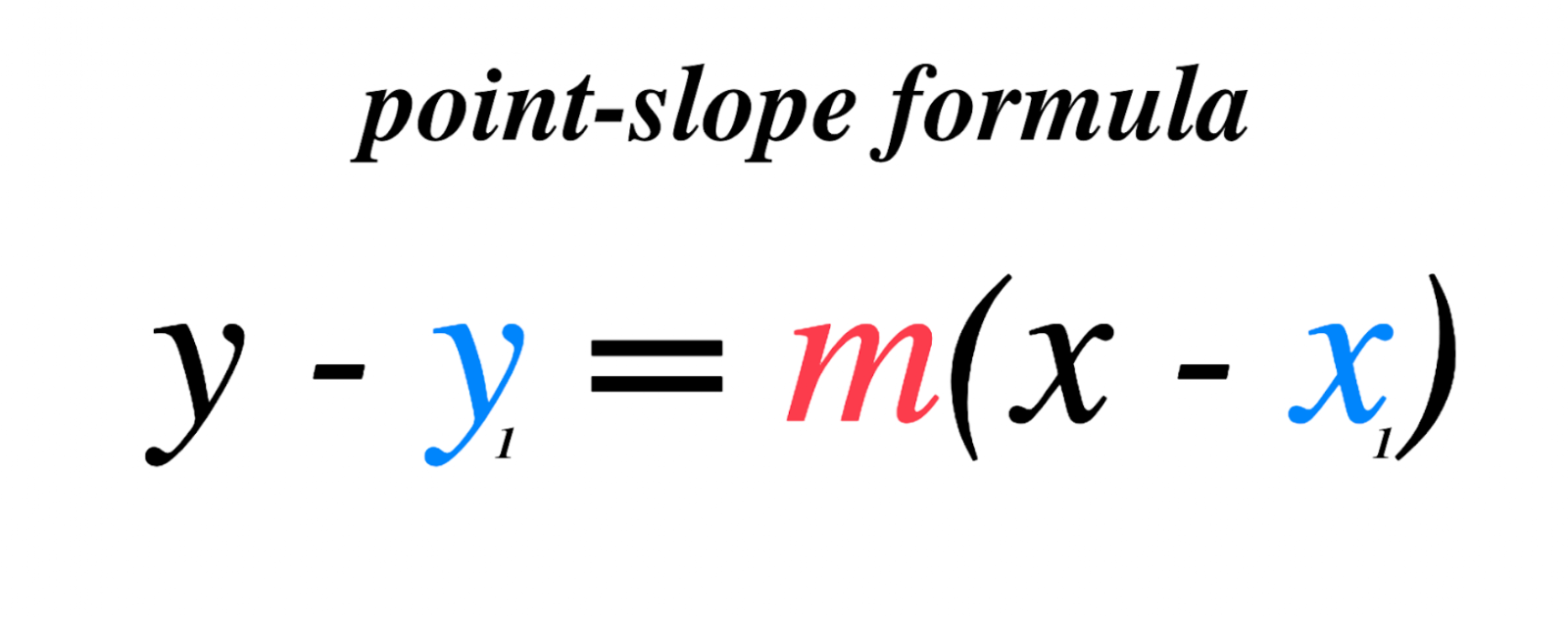Creating an equation of a line when given 2 points.

In order to find a linear equation when 2 points are given, you need to follow these TWO steps:

Step 1 ➡️ You need to calculate a slope (m)

Step 2 ➡️ Plug in one point (x,y) for x and y to solve for b

Step 1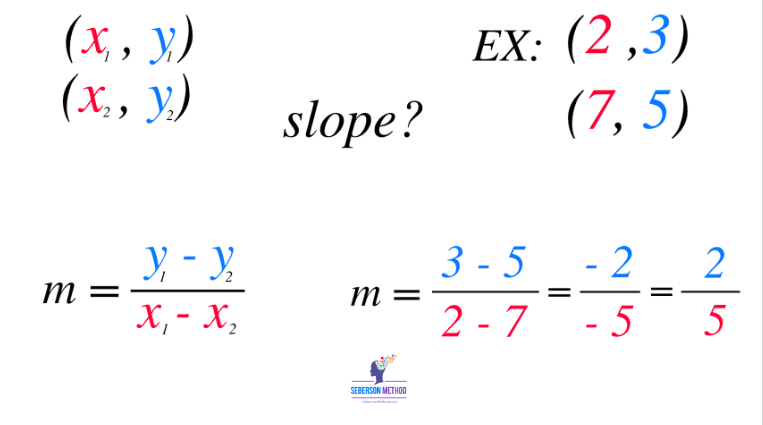Sometimes, the SAT will hand you the coordinates of the two points; other times, you’ll have to work to recognize them. Here, they give you the points right away.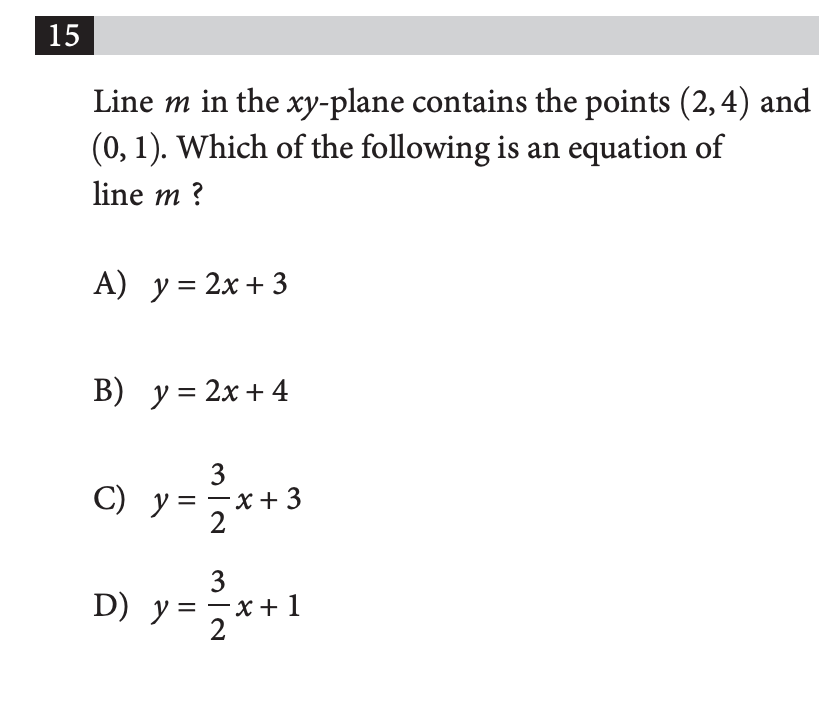Please find a written out solution below⬇️⬇️⬇️⬇️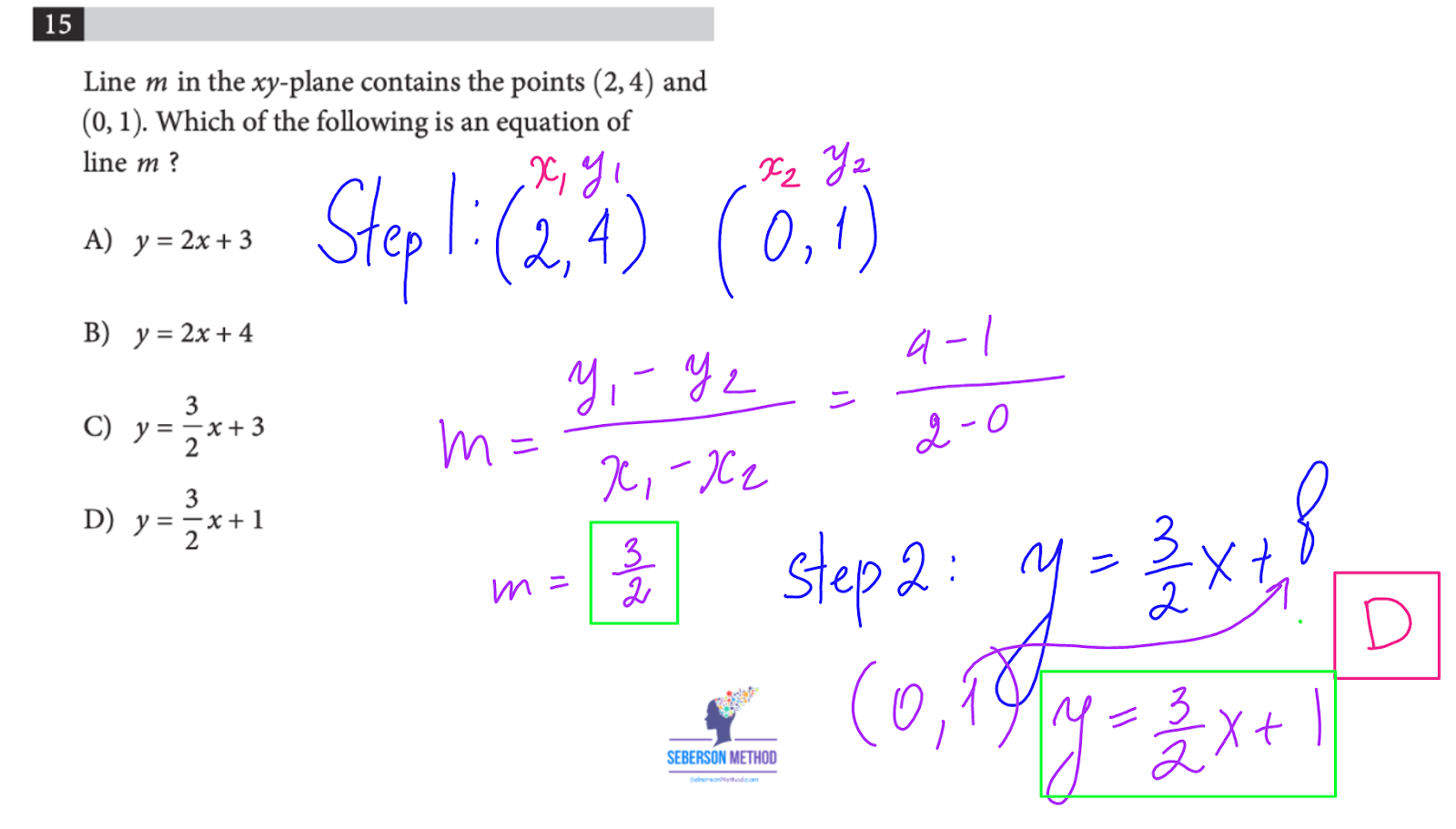Other times, you have to work to find your points. Sometimes, you’ll have to get them from a graph or a table.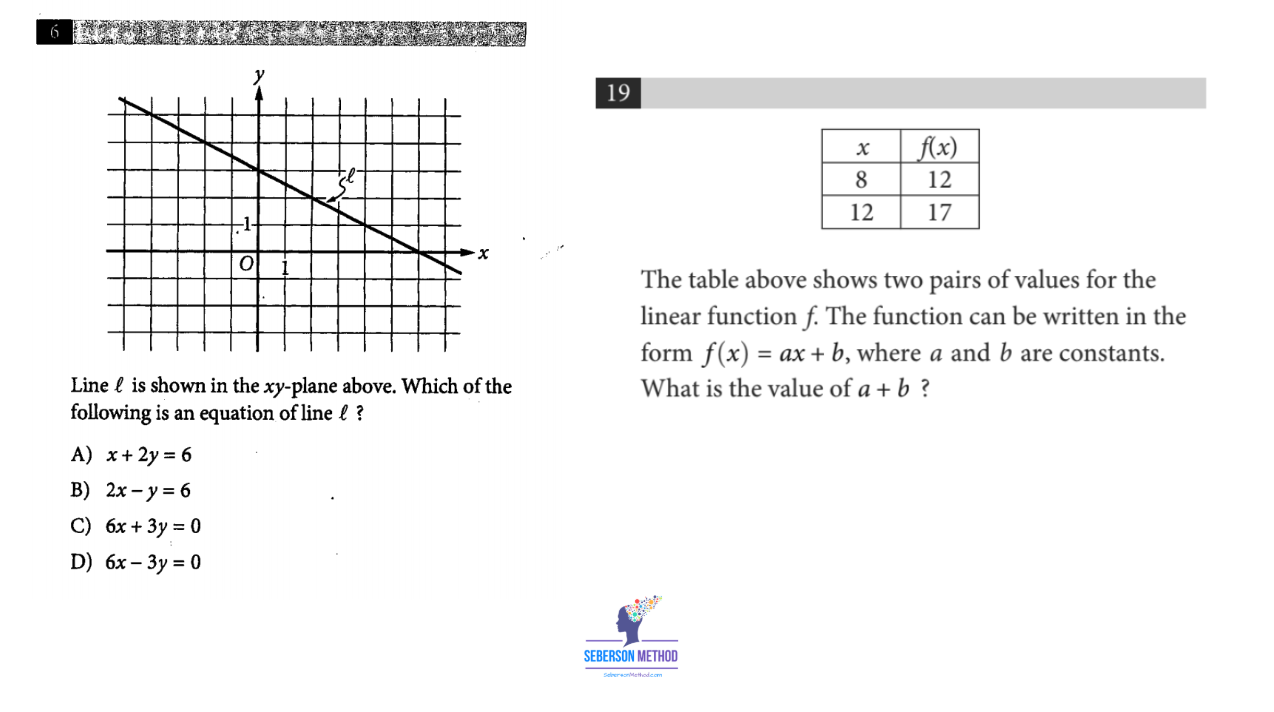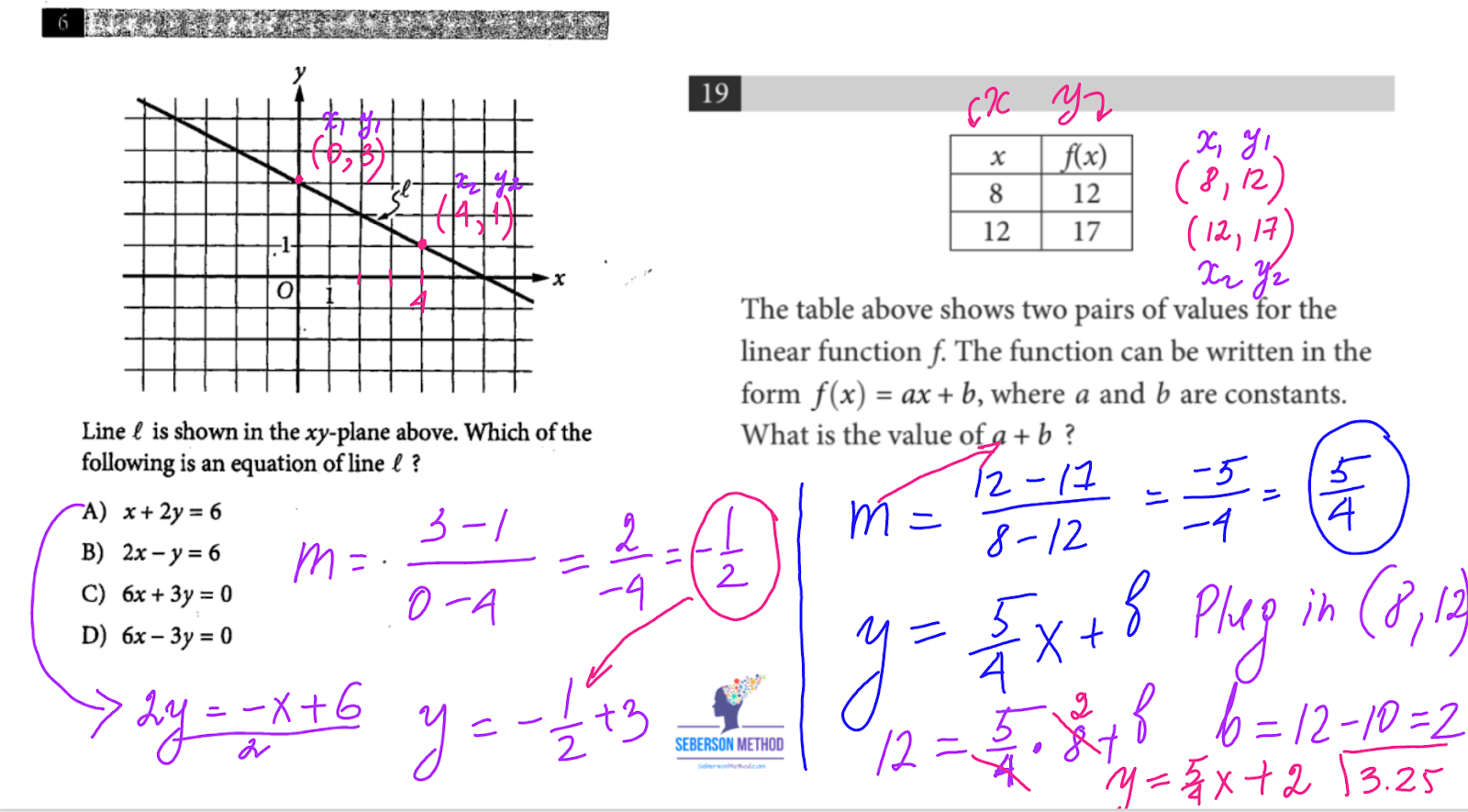If f(x) scares or confuses you, remember that f(x) is a function notation, and - more importantly - it’s the same thing as your y value.

So if you see a g(x) or f(x), joyfully cross it out and replace it with y.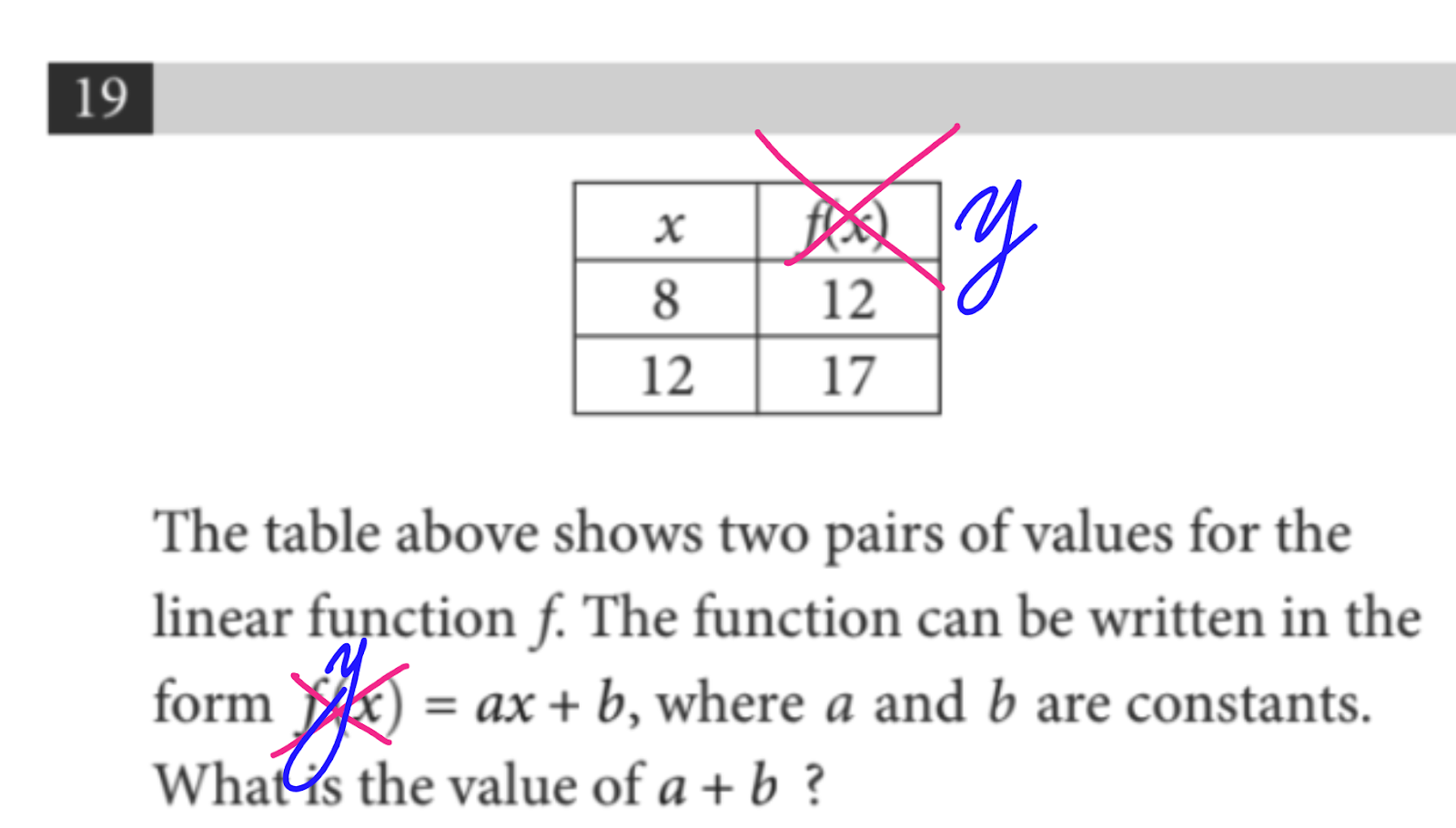One more thing,

Please keep in mind that the expression f(6) = 7 actually gives you a coordinate (6, 7)Parallel and Perpendicular Lines

The concept of parallel and perpendicular lines is one of the most frequently tested on the SAT and ACT.

🔵If two lines are parallel, they have the same slope (m).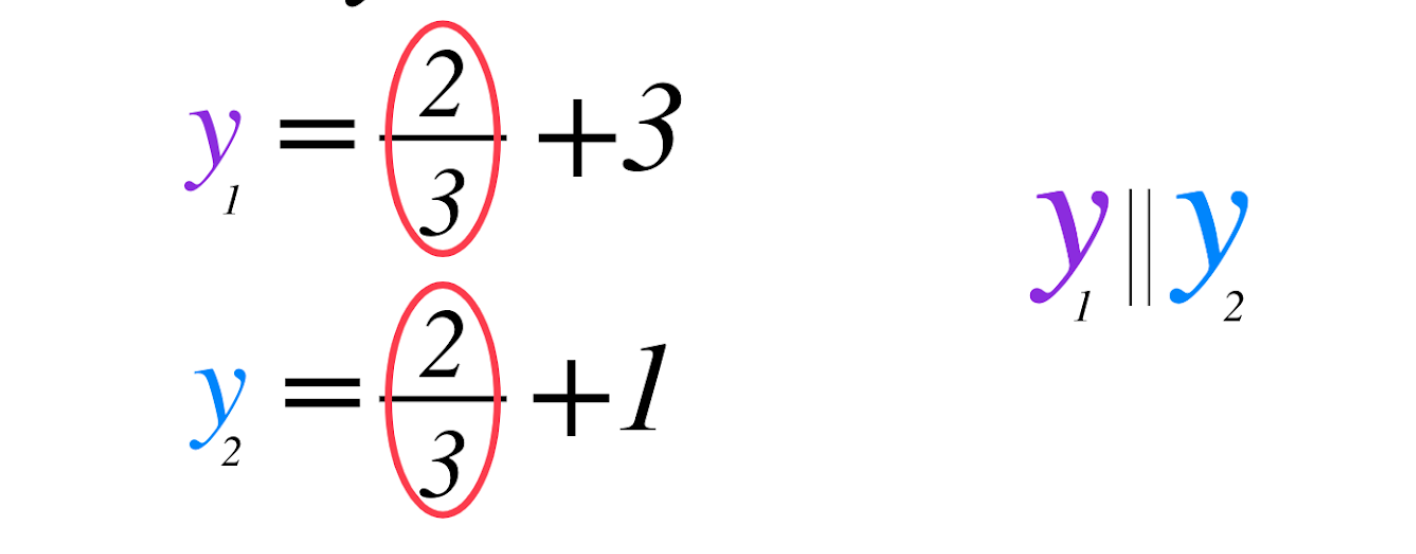🔵If two lines are perpendicular, their slopes are negative reciprocals of each other.

What’s a negative reciprocal? 😡In order to efficiently use your time, it helps to know these two tips:

Tip # 1 ➡️ A straight horizontal line has a slope of zero.

Tip # 2 ➡️ A straight vertical line has an undefined slope (because the run will always be 0, and you can't divide by 0).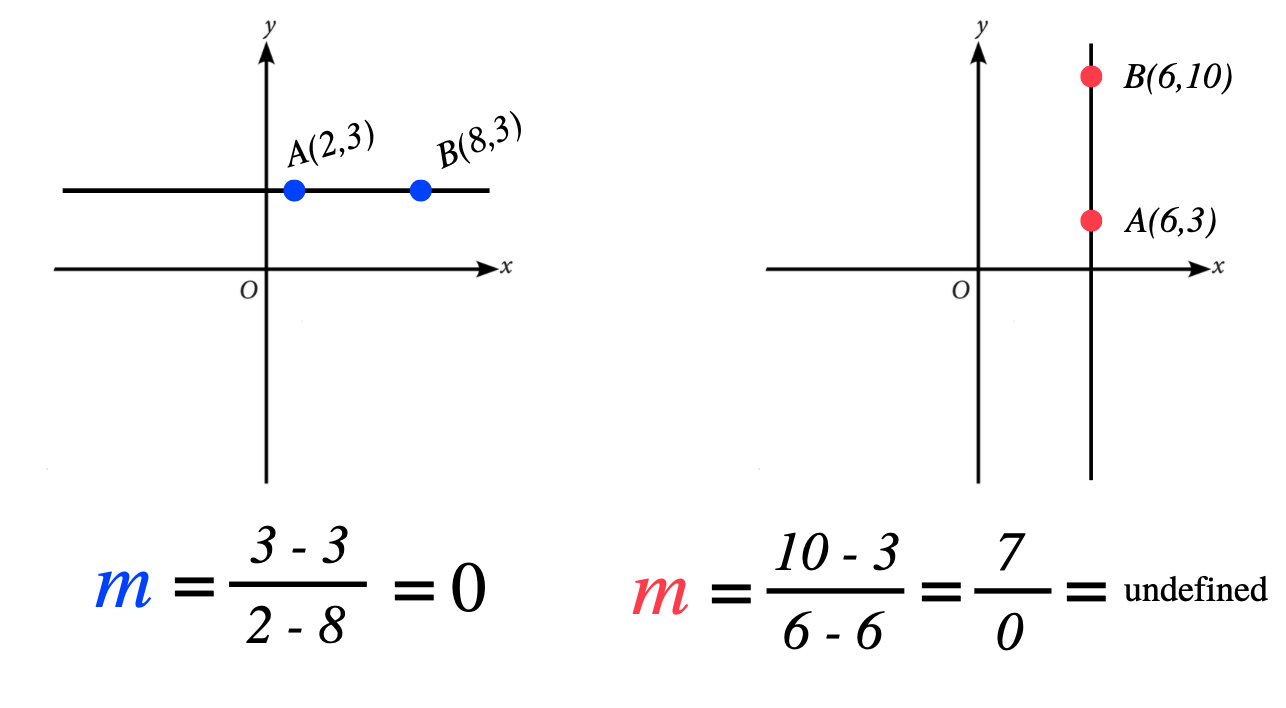Finding X and Y intercepts.

The SAT will ask you - directly or indirectly - to locate your x and y-intercepts a few times during the test.

🔴To find an x - intercept, plug in 0 for y variable.

🔵To find y - intercept, plug in 0 for x variable.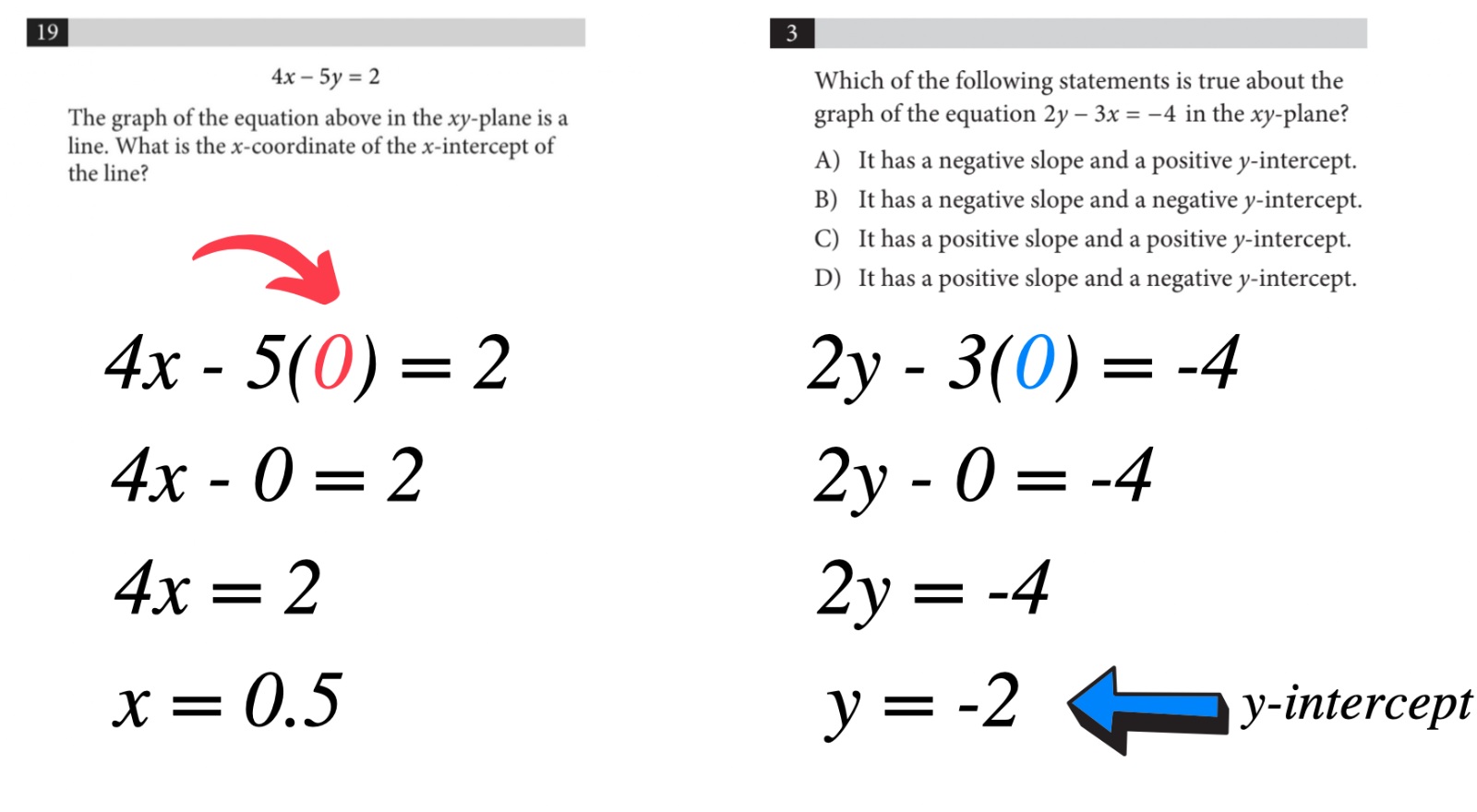If you'd rather learn this from a video, please click on this link to be rediracted to a video tutorial.